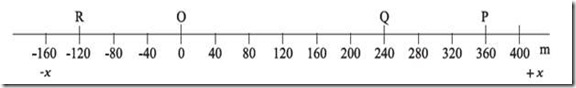# Physics XI | Frame of Reference

## Frame of Reference:

Suppose someone asked you a question “How far is India?” your reaction would be “from where?” Then the person who asked you the question would tell, from here or from United States. So in kinematics some reference is very necessary to measure distances.

Suppose, you are sitting inside a stationary train. At the same time other train is moving. It is very hard to tell which of the trains is moving. If you consider yourself stationary, other train is moving while for the passenger of the other train, your train is moving.

Same is true in case of ground. For a person standing on the ground, you are moving while sitting in the train. While for the passengers sitting in the train, you are stationary.

So motion and rest are related terms depending on the position of the observer.

One Dimensional frame of Reference:

Suppose you have to locate a point (Point P as shown in figure) on a line without touching it. The solution is to mark the line and mark one point as zero (point ‘O’ in figure) and measure the distance of the point ‘P’ from the zero point. In our case the distance of P from the point is 360m.So we always need a ‘reference point’ from which all the distances would be measured. Same is true with the 2-Dimensional motion. In the case of 2 Dimensional motion, we need a system of coordinate axes to explain the motion.

The definition of the Reference Frame is “A system of coordinate axes which defines the position of a particle or an event in two or three dimensional space is called a Frame of Reference”.

This site uses Akismet to reduce spam. Learn how your comment data is processed.